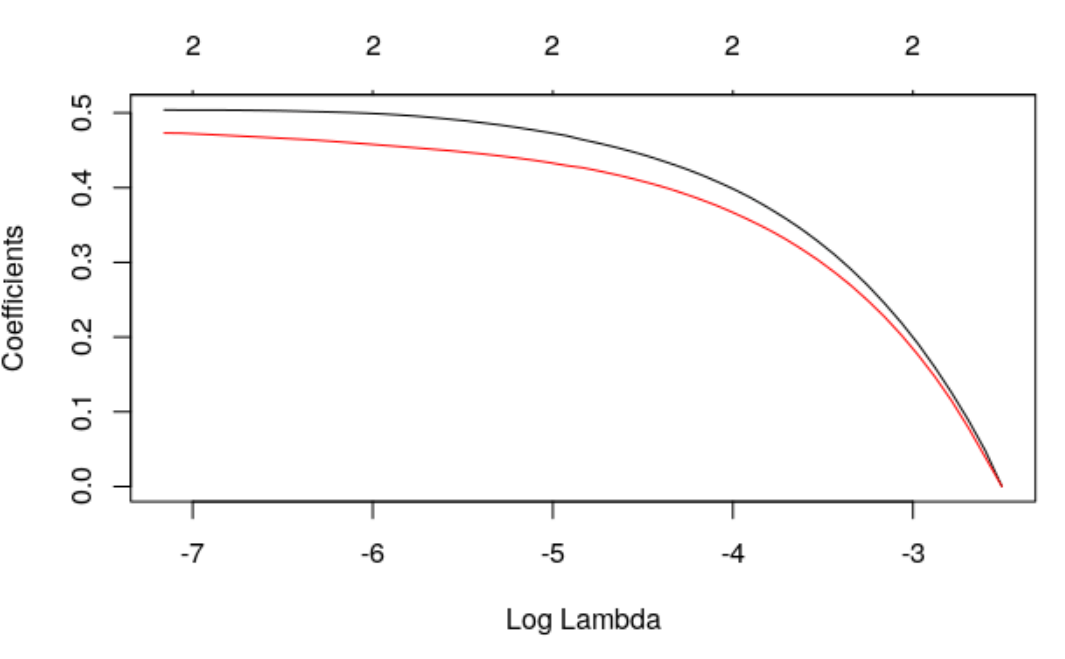Want to share your content on R-bloggers? click here if you have a blog, or here if you don't.

There are important discussions nowadays about data modeling, to choose between the “two cultures” (as mentioned in Breiman (2001)), i.e. either econometrics models or machine/statistical learning models. We did discuss this issue recently in Econométrie et Machine Learning (so far only in French) with Emmanuel Flachaire and Antoine Ly. One argument often used by econometricians is the interpretability of econometric models. Or at least the attempt to get an interpretable model.

We also have this discussion in actuarial science, for instance in ratemaking (or insurance pricing). Machine learning based models usually perform better (for some a priori chosen metric), but actuaries claim that econometric models are more easily interpretable. In actuarial literature, we assume that claim frequency [latex]Y[/latex] is driven by some non-observable risk factor [latex]\Theta[/latex], and therefore, we do have heterogeneous risks in our portfolio. And, it can be seen as legitimate to differentiate prices. Assume that this risk factor [latex]\Theta[/latex] is strongly correlated with [latex]X_1[/latex], the age of the driver. Because in our portfolio, old drivers tend to have more accidents. Here, we could pretend to have a “causal story” (as defined in Freedman (2009)) because of a possible interpretation of the model. So it is natural here to consider a regression model of [latex]Y[/latex] on [latex]X_1[/latex] to derive our actuarial pricing model. But assume that, possibly, risk factor [latex]\Theta[/latex] is also strongly correlated with [latex]X_2[/latex], that can be related to spatial features (say latitude, which denoted a north/south position). Because in our portfolio, drivers living in the south tend to have more accidents (reads are known to be more dangerous there). Here, we could pretend to have a second “causal story”.

Of course, since [latex]\Theta[/latex] is strongly correlated with [latex]X_1[/latex] and [latex]X_2[/latex], it means that [latex]X_1[/latex] and [latex]X_2[/latex] are strongly correlated. Here also, this correlation can be interpreted (not in a causal way as previously, but still), since we know that old people like to live in southern regions. So, what should we do here ? Let us run some simulations to  illustrate.

``` set.seed(123)
n=1e5
Theta=rnorm(n)
X1=Theta+rnorm(n)/8
X2=Theta+rnorm(n)/8
L=exp(-3+Theta)
Y=rpois(n,L)
B=data.frame(Y,X1,X2)```

Our first idea was to consider a model where [latex]Y[/latex] is “explained” by the first variable [latex]X_1[/latex],

``` g1=glm(Y~X1,data=B,family=poisson)
summary(g1)

Coefficients:
Estimate Std. Error z value Pr(>|z|)
(Inter.) -2.97778    0.01544 -192.88   <2e-16 ***
X1        0.97926    0.01092   89.64   <2e-16 ***```

As expected, our variable is “significant”, but also, probably more interesting, [latex]X_2[/latex], has no impact on the residuals

``` B\$e1=residuals(g1,type="pearson")
g1e=lm(e1~X2,data=B)
summary(g1e)

Coefficients:
Estimate Std. Error t value Pr(>|t|)
(Inter.) 0.0003618  0.0031696   0.114    0.909
X2       0.0028601  0.0031467   0.909    0.363```

The interpretation is that once we corrected claim frequency for the age of the drivers, there is no spatial effect here. So, a good model should be based only on the age of the drivers.

But we can also consider the other story. We can consider a model where [latex]Y[/latex] is “explained” by the second variable [latex]X_2[/latex],

``` g2=glm(Y~X2,data=B,family=poisson)
summary(g2)

Coefficients:
Estimate Std. Error z value Pr(>|z|)
(Inter.) -2.97724    0.01544 -192.81   <2e-16 ***
X2        0.97915    0.01093   89.56   <2e-16 ***```

Here also we have a valid model, that can be interpreted, and here also [latex]X_1[/latex], has no impact on the residuals

``` B\$e2=residuals(g2,type="pearson")
g2e=lm(e2~X1,data=B)
summary(g2e)

Coefficients:
Estimate Std. Error t value Pr(>|t|)
(Inter.) 0.0004863  0.0031733   0.153    0.878
X1       0.0027979  0.0031504   0.888    0.374```

The story is similar here. If we correct from the spatial pattern, claims frequency does not depend on the age of the driver.

So, what should we do now? We do have two models, and each of them is as interpretable as the other one. Note that we can not use any statistical tool to distinguish the two: they are comparable

``` AIC(g1)
 51013.39
AIC(g2)
 51013.15```

Why not incorporate the two explanatory variables [latex]X_1[/latex] and [latex]X_2[/latex], at the same time, in our regression model, and let “the model” decide what to do…?

``` g=glm(Y~X1+X2,data=B,family=poisson)
summary(g)

Coefficients:
Estimate Std. Error  z value Pr(>|z|)
(Inter.) -2.98132    0.01547 -192.723    2e-16 ***
X1        0.49310    0.06226    7.920 2.38e-15 ***
X2        0.49375    0.06225    7.931 2.17e-15 ***```

It looks like we completely lost the interpretability of the model, since our two explanatory variables are (strongly) correlated. Actually, instead of saying “use one, and drop the other one (since it brings no further information)”, it says “use both, each one will explain half of the variable”. Strange interpretation, isn’t it?  So why not try some LASSO here?

```library(glmnet)
fit=glmnet(x=as.matrix(B[,c("X1","X2")]),
y=B\$Y,family="poisson")
plot(fit,xvar="lambda")```Here also, it says that we either keep both, or none. So it cannot be used for variable selection (which is an important motivation to use LASSO technique). So, what should be do if we several interpretable models, but no way to choose? Because usually, we claim that we prefer to use a model with an interpretation. But what should be done here?